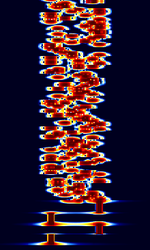# RTTYM

```OLIVIA based Modes: Olivia - Contestia - RTTYM - PAX - VOICE
```

RTTYMFrequencies 3 MHz,30 MHz
Frequency Range 3 MHz - 30 MHz
Mode USB
Modulation MFSK
ACF
Emission Designator
Bandwidth 150 Hz,250 Hz,500 Hz,1000 Hz,2 kHz
Location Worldwide
Short Description RTTYM, developed by Nick Fedoseev (UT2UZ) in 2005, is a digital mode derived from Olivia. It aims to deliver a compromise of speed and performance. RTTYM is about 4x faster than Olivia, but trades the speed for reduced robustness and sensitivity.
I/Q Raw Recording
Audio Sample

RTTYM, developed by Nick Fedoseev (UT2UZ) in 2005, is a digital mode derived from Olivia. It aims to deliver a compromise of speed and performance. RTTYM is about 4x faster than Olivia, but trades the speed for reduced robustness and sensitivity.

Compared to Olivia, RTTYM has +3 dBThe decibel (dB) is a logarithmic unit used to express the ratio of two values of a physical quantity, here the strength of a received signal. minimum signal to noise ratio compared to Olivia. RTTYM uses a block size of 16 where Olivia uses a block size of 64. RTTYM uses a slightly modified RTTY Baudot 5-bit alphabet encoding.

## RTTYM Submodes

125, 250, 500, 1000, and 2000 are the available bandwidths. 4, 8, 16, 32, 64, 128, and 256 are the available tones. There are a total of 40 possible submodes.

RTTYM's submodes are determined by bandwidth and number of tones. This is represented with the "RTTYM X/Y" or "RTTYM X-Y" format, where X = # of tones and Y = Bandwidth in HzHertz (Hz), unit of frequency, defined as one cycle per second (1 Hz).. Y/X is also alternatively used. E.g: RTTYM 8-250 = 8/250 = 250/8 = 8 tones, 250 HzHertz (Hz), unit of frequency, defined as one cycle per second (1 Hz). bandwidth.

The standard RTTYM submodes (bandwidth/tones) are 4/125, 8/250, 16/500, 32/1000, and 64/2000.

The most commonly used submodes right now are 8/125, 4/250, 8/250, 4/500, 8/500, 16/500, 8/1000, 16/1000 and 32/1000.

 2/125 4/125 8/125 16/125 32/125 64/125 128/125 256/125 2/250 4/250 8/250 16/250 32/250 64/250 128/250 256/250 2/500 4/500 8/500 16/500 32/500 64/500 128/500 256/500 2/1000 4/1000 8/1000 16/1000 32/1000 64/1000 128/1000 256/1000 2/2000 4/2000 8/2000 16/2000 32/2000 64/2000 128/2000 256/2000

## Mode Characteristics

• 8-250: "Slow" 8 tones, bandwidth=250 HzHertz (Hz), unit of frequency, defined as one cycle per second (1 Hz)., speed=31.25 bauds, 58 wpm, lowest S/N=-12 dBThe decibel (dB) is a logarithmic unit used to express the ratio of two values of a physical quantity, here the strength of a received signal.,
• 16-500: "Average" 16 tones, bandwidth=500 HzHertz (Hz), unit of frequency, defined as one cycle per second (1 Hz)., speed=31.25 bauds, 78 wpm, lowest S/N =-10.5 dBThe decibel (dB) is a logarithmic unit used to express the ratio of two values of a physical quantity, here the strength of a received signal.,
• 32-1000: ("Standard" default mode) 32 tones, bandwidth=1000 HzHertz (Hz), unit of frequency, defined as one cycle per second (1 Hz)., speed=31.25 bauds, 97 wpm, lowest S/N =-10 dBThe decibel (dB) is a logarithmic unit used to express the ratio of two values of a physical quantity, here the strength of a received signal.,
• 8-500: "Normal" 8 tones, bandwidth=500 HzHertz (Hz), unit of frequency, defined as one cycle per second (1 Hz)., speed=62.5 bauds, 117 wpm, lowest S/N =-9 dBThe decibel (dB) is a logarithmic unit used to express the ratio of two values of a physical quantity, here the strength of a received signal.,
• 16-1000: "Fast" 16 tones, bandwidth=1000 HzHertz (Hz), unit of frequency, defined as one cycle per second (1 Hz)., speed=62.5 bauds, 156 wpm, lowest S/N =-7.5 dBThe decibel (dB) is a logarithmic unit used to express the ratio of two values of a physical quantity, here the strength of a received signal.,
• 4-500: "Fast 2" 4 tones, bandwidth=500 HzHertz (Hz), unit of frequency, defined as one cycle per second (1 Hz)., speed=125 bauds, 156 wpm, lowest S/N =- 6 dBThe decibel (dB) is a logarithmic unit used to express the ratio of two values of a physical quantity, here the strength of a received signal..
• 4-250: "Average 2" 4 tones, bandwidth=250 HzHertz (Hz), unit of frequency, defined as one cycle per second (1 Hz)., speed=62,5 bauds, 78 wpm, lowest S/N =-8.5 dBThe decibel (dB) is a logarithmic unit used to express the ratio of two values of a physical quantity, here the strength of a received signal.,
• 8-1000: "Very fast" 8 tones, bandwidth=1000 HzHertz (Hz), unit of frequency, defined as one cycle per second (1 Hz)., speed=125 bauds, 234 wpm, lowest S/N =-3 dBThe decibel (dB) is a logarithmic unit used to express the ratio of two values of a physical quantity, here the strength of a received signal..

## Decoding Software

Hobby Level Software